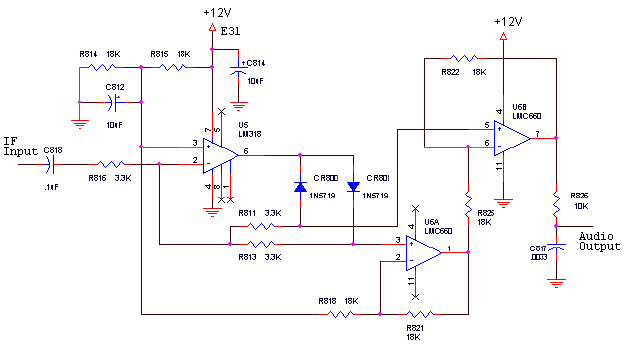An improved, precision, full wave AM detector.

By Rob Schenck, K2CU   K2CU@arrl.net

Detector SchematicTheory of Operation

This circuit is a variation of the classic "Precision Half Wave Rectifier" as described in many op-amp application notes as shown below:Its operation is based upon basic principles of operational amplifier circuits. An ideal op-amp has extremely high input impedance, extremely low output impedance, and extremely high gain. Note the operative word "extremely". The non inverting input (+) of the op-amp is connected to ground. Feedback is provided by the two paths R2+D1 and D2. The op-amp will drive its output pin to a voltage such that the inverting input (-) is at ground voltage. There will be a slight variation (a few millivolts) from 0 volts as a result of what is known as input offset voltage, a property of real op-amps. The inverting input is often called the summing junction, as the sum of the currents in that node will be zero. Since virtually no current flows into the + input of the op-amp itself, any current coming in through the input resistor R1 will be met by an equal and opposite current coming through either of the two paths of R2+D1 or D2.

The I.F. input signal will produce an input current of:

Iin = Vin/R1